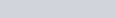ChemTalk

# What is Ka, the acid dissociation constant?## Core Concepts

In this tutorial you will learn about the acid dissociation constant (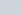), and its applications to other topics in chemistry. You will learn how to relateand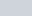as well as the relationship betweenand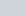.

## What is the acid dissociation constant (Ka)?, or the acid dissociation constant is used to differentiate strong and weak acids. If an acid dissociates more the value forwill decrease and vice versa if the acid is less likely to dissociate in water, thevalue will be less. So, for example a strong acid like hydrochloric acid (HCl) will have a higher dissociation constant,, than the weak acid hydroflouric acid (HF). This is because weak acids are less likely to ionize in water, they exist mostly as molecules, while strong acids will exist as mostly ions in water.

Theis also the equilibrium constant of an acid’s dissociation reaction. This is a quantitative value for the strength of an acid in solution. The acid dissociation constant has no units.is equal to the products over the reactants.

#### Steps to Calculating Ka

Here are the steps to calculate:

1. Take the product of the concentrations of the dissociated ions.
2. Then divide this number by the concentration of your starting acid. An easy way to remember this is “products over reactants”.

Ex.The brackets around the hydrogen ion, bromine ion, and hydrobromic acid indicate molarity. So in order to find a quantitative value for theof this reaction, we would need to have the molarities of each of these items.

In essence, the higher the value for, the more the acid will dissociate. The more an acid dissociates, the stronger the acid; the stronger the acid, the higher thevalue. In contrast, weaker acids will generally have lowervalues than stronger acids because weak acids only partially disassociate meaning that there are more reactants versus products.

#### Equilibrium value (K value)

The equilibrium value of a reaction will depict the ratio between the products of the reaction divided by the reactants. If a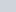value for a reaction is high, the reaction will favor the products and will proceed in the foreword direction. Similarly, if thevalue of the reaction is low, the reaction will favor the reactants and proceed in the backward direction.

## Acid-base reactions

Theof an acid-base reaction can be calculated if one knows thevalues of the reactants and products. Raise 10 to the power of the difference invalues in the conjugate acid and reactant acid.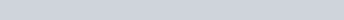If the reaction has a weak conjugate acid and a strong reactant acid, the equilibrium constant will be high. This means that the reaction will be driven mostly to completion. Conversely, if an acid-base reaction has a strong acid as its conjugate acid, the equilibrium constant would be incredibly low.

## Calculating pH from Acid Dissociation Constants

The acid dissociation constant value can be used in a lot of different scenarios, in some cases it can be used to calculate the. Let’s use the example of a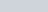solution of HBr.

• Our first step should be to write the chemical reaction for the ionization that forms H+ ions –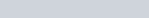• The second step is to write the equilibrium expression for this reaction, which is the products over the reactants.• Next, we should use our knowns to create an ICE chart to solve for the concentration of the hydrogen ion.
• After creating the ICE chart we need to use these values to define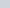. Use the equilibrium expression above plug in your values and solve for.. This will give us a value for the concentration of the hydrogen ion.
• Finally, since the formula foris equal to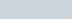we find that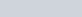.

## Relating pKa and Ka Acid Dissociation Values

Theand thevalues are usually most useful when determining to what degree an acid will ionize in a solution. Like the acid dissociation constant,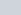is the base dissociation constant. The acid dissociation constant and the base dissociation constant are related through the ion constant for water,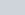.

So,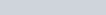Anytime we see the lower case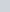in front of a constant in chemistry, it will usually mean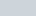of that value. Since we know thatis the acid dissociation constant,simply just meansof the acid dissociation constant value. Acids will usually dissociate according to the following equations,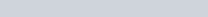(The letter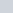denotes acid)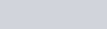here we can see the products of the reaction over the reactants.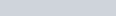(note that in the previous question we obtained a value forso, now we can find the value for).

You may notice from before that a strong acid will produce a larger acid dissociation constant value because acid is almost completely dissociated. Since, the ionized form of the acid is favored in the dissociation of a strong acid, the reaction will favor proceeding to the left (the side of the reactions).can tell us the same thing just in the inverse order. So, a smallerwill show a stronger acid.

## Acid Dissociation Constant Practice Problems

Problem 1

Does a highervalue indicate a weaker or a stronger acid?

Problem 2

Phenylacetic acid reacts with hydroxide (the conjugate base of water) according to the following reaction equation: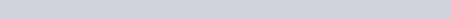Phenylacetic acid has aof 4.28. What is theof the reaction at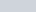(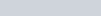?

## Acid Dissociation Constant Practice Problem Solutions

1: Weaker

2: